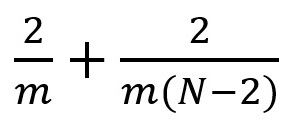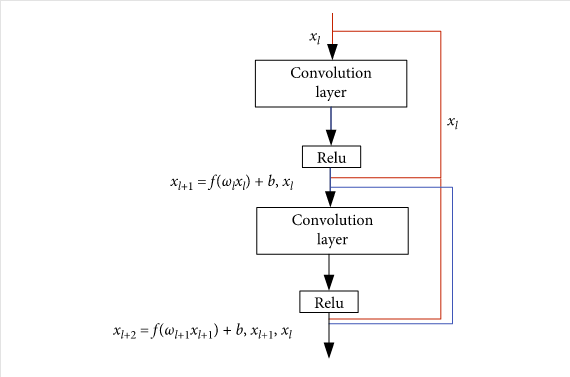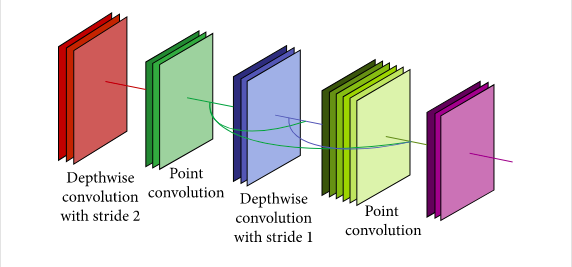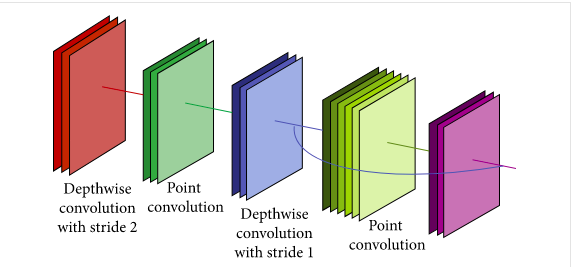# Transfer Learning — Part — 6.0!! Mobile net

In Part 5 Series of the Transfer Learning series we have discussed the Residual Nets in depth along with hands-on application of these pre-trained neural nets in Keras and PyTorch API’s. The datasets on which these pre-trained model is trained for the ILVRC competition which is held annually and their repository as well as the documentation in order to implement this concept with two API’s namely Keras and PyTorch which is discussed in Part 5 of this series. In this, article we will discuss theoretically about the Residual Nets and in article 6.2 and 6.3 we will have practical implementation with Keras and PyTorch API respectively. The link of notebook for setting up the along with the article is given below:

For the repository and document please follow below two mentioned links:

Keras:

PyTorch:

## 1. History of Mobilenet

Numerous models are coming into existence, big thanks to Imagenet competition ILRVSC competition. Earlier opencv algorithms like SHIFT, HOG, LBPH and many other have performed well but their feature set was constructed manually and they lacks the capability of self learning thought they consumed less resources. As the popularity of the ILRVSC competition plummeted, size, efficiency as well as the real-time use of these model also increases.

The rise of the huge and cheap computational power enable these model to successfully run on these high power compute. But if we look into other side of the coin everyone does not have these kind of computational technology and when it comes to deployment and real time use we have limited resources especially in user end devices. So the deployment of the model was not possible at user end due to huge number of parameters and the the computation it requires to optimisation, forward propagation and back propagation.

Mobilenet solved the problem of huge parameters by employing seperable convolutional technique and super imposing the feature maps from the previous layer in forward convolutional layer. In order to further reduce the number of network parameters and improve the classification accuracy, dense blocks that are proposed in DenseNets are introduced into MobileNet. In Dense-MobileNet models, convolution layers with the same size of input feature maps in MobileNet models are taken as dense blocks, and dense connections are carried out within the dense blocks. The new network structure can make full use of the output feature maps generated by the previous convolution layers in dense blocks, so as to generate a large number of feature maps with fewer convolution cores and repeatedly use the features. By setting a small growth rate, the network further reduces the parameters and the computation cost. Two Dense-MobileNet models, Dense1-MobileNet and Dense2-MobileNet, are designed. Experiments show that Dense2-MobileNet can achieve higher recognition accuracy than MobileNet, while only with fewer parameters and computation cost. In order to further reduce the number of network parameters and improve the classification accuracy, dense blocks that are proposed in DenseNets are introduced into MobileNet. In Dense-MobileNet models, convolution layers with the same size of input feature maps in MobileNet models are taken as dense blocks, and dense connections are carried out within the dense blocks. The new network structure can make full use of the output feature maps generated by the previous convolution layers in dense blocks, so as to generate a large number of feature maps with fewer convolution cores and repeatedly use the features. By setting a small growth rate, the network further reduces the parameters and the computation cost. Two Dense-MobileNet models, Dense1-MobileNet and Dense2-MobileNet, are designed. Experiments show that Dense2-MobileNet can achieve higher recognition accuracy than MobileNet, while only with fewer parameters and computation cost.In order to further reduce the number of network parameters and improve the classification accuracy, dense blocks that are proposed in DenseNets are introduced into MobileNet. In Dense-MobileNet models, convolution layers with the same size of input feature maps in MobileNet models are taken as dense blocks, and dense connections are carried out within the dense blocks. The new network structure can make full use of the output feature maps generated by the previous convolution layers in dense blocks, so as to generate a large number of feature maps with fewer convolution cores and repeatedly use the features. By setting a small growth rate, the network further reduces the parameters and the computation cost. Two Dense-MobileNet models, Dense1-MobileNet and Dense2-MobileNet, are designed. Experiments show that Dense2-MobileNet can achieve higher recognition accuracy than MobileNet, while only with fewer parameters and computation cost. Compared with VGG-16 network, MobileNet is a lightweight network, which uses depthwise separable convolution to deepen the network, and reduce parameters and computation. At the same time, the classification accuracy of MobileNet on ImageNet data set only reduces by 1%. However, in order to be better applied to mobile devices with limited memory, the parameters and computational complexity of the MobileNet model need to be further reduced. Therefore, we use dense blocks as the basic unit in the network layer of MobileNet. By setting a small growth rate, the model has fewer parameters and lower computational cost. The new models, namely Dense-MobileNets, can also achieve high classification accuracy. MobileNet is a streamlined architecture that uses depthwise separable convolutions to construct lightweight deep convolutional neural networks and provides an efficient model for mobile and embedded vision applications.

Lets start this article by explaining how the convolution works then we will dig deep into seperable convolution layers.

1. Convolution Layers

For images/video the best way to extract the features is by convoluting the filters/kernels which is of 2d dimension and extract the different feature maps from the image. A 2D Convolution is a mathematical process in which a 2D kernel slides over the 2D input matrix performing matrix multiplication with the part that is currently on and then summing up the result matrix into a single pixel.

Suppose there is an input data of size Df x Df x M, where Df x Df can be the image size and M is the number of channels (3 for an RGB image and 1 for GreyScale). Suppose there are N filters/kernels of size Dk x Dk x N. If a normal convolution operation is done, then, the output size will be Dp x Dp x N.

## Calculations for convolution:

No of multiplication in 1 convolution operation = Dk * Dk * M (eq 1)

Since, we have N filters and each filter slides vertically and horizontally Dp times so,

Total number of multiplication = N * Dp * Dp * (eq.1 )(Multiplications per convolution) (eq. 2)

if we calculate using eq 1 and eq 1 we can infer that

Total multiplication =N *Dp* Dp*Dk*Dk *M (eq. 3)

These calculation are very huge in number and need substantial resource to compute the model and optimise it. In order to save the computing resources and make the model which can be deployed in the real time devices researchers developed concept known as separable convolution in which we can separate the kernels/filters in to smaller kernal for reducing the size of the kernels which directly reduces the size of the computation and prevents over fitting.

2. Separable Convolution

A Separable Convolution is a process in which a single convolution can be divided into two or more convolutions to produce the same output. A single process is divided into two or more sub-processes to achieve the same effect. Let’s understand Separable Convolutions, their types in-depth with examples.

Mainly there are two types of Separable Convolutions

2.1 Spartial Seperable Convolution

This is the easier one out of the two, and illustrates the idea of separating one convolution into two well, so we start with this. Unfortunately, spatial separable convolutions have some significant limitations, meaning that it is not heavily used in deep learning because spartial refers to height and weight of the image it does not take account of the depth .The spatial separable convolution is so named because it deals primarily with the spatial dimensions of an image and kernel: the width and the height. (The other dimension, the “depth” dimension, is the number of channels of each image).

The spatial separable convolution works on the spatial features of an image: its width and its height. These features are the spatial dimensions of the image hence the name, spatial separable. It decomposes the convolution operation into two parts and applies each separated convolution in succession. For instance, the Sobel filter (or the Sobel kernel), which is a 3x3 filter is split into two filters of size 3x1 and 1x3.

As you can see, the 3x3 filter is spilt into a 3x1 and a 1x3 filter. The output does not change as the image still obeys the matrix multiplication rule. The image when the 3x1 filter is applied will have the column dimension as 1 which is the row dimension of the next filter, the 1x3 filter. What is changed is the number of multiplications that are performed. Spatial separable convolution reduces the number of individual multiplications. In a regular 3x3 convolution, there are a total of 9 operations. But when we split the matrix into a 3x1 and a 1x3 filter, there are a total of 6 operations. Therefore, less matrix multiplications are needed when convolving it in an image.

Let’s generalize the formula. For any N x N image, applying convolutions with an m x m kernel having stride of 1 and padding of 0:

Traditional convolution requires ((N — 2) x (N — 2) x m x m) matrix multiplications. (eq. 4)

Spatially separable convolution requires (N x (N-2) x m) + ((N-2) x (N-2) x m) = (2N-2) x (N-2) x m multiplications. (eq. 5)

We can find the ratio of computation costs between both the approaches. The ratio between computational cost of spatial separable convolution and computational cost of regular convolution is:Fig . 5 Ration of CNN and Spartial CNN

We can see this ratio becomes 2 / m when the image size is way larger than the filter size (when N >> m). Putting values of kernel size, m = 3, 5, 7, and so on, we see that the computational cost of spatially separable convolution is 2/3 (about 66%) of the standard convolution for a 3 x 3 filter, 2 / 5 (40%) for a 5 x 5 filter, 2 / 7 (about 29%) for a 7 x 7 filter.

An important thing to note here is that not every kernel can be separated. Because of this drawback, this method is used lesser compared to Depthwise separable convolutions.

2.2 Depth wise Separable Convolution

Unlike spatial separable convolutions, depthwise separable convolutions work with kernels that cannot be “factored” into two smaller kernels. Hence, it is more commonly used. This is the type of separable convolution seen in tf.keras.layers.SeparableConv2D or tf.layers.separable_conv2d.

The depthwise separable convolution is so named because it deals not just with the spatial dimensions, but with the depth dimension — the number of channels — as well. An input image may have 3 channels: RGB. After a few convolutions, an image may have multiple channels. You can image each channel as a particular interpretation of that image; in for example, the “red” channel interprets the “redness” of each pixel, the “blue” channel interprets the “blueness” of each pixel, and the “green” channel interprets the “greenness” of each pixel. An image with 64 channels has 64 different interpretations of that image.

Similar to the spatial separable convolution, a depthwise separable convolution splits a kernel into 2 separate kernels that do two convolutions: the depth wise — for spartial operation convolution and the point wise convolution — for depth wise operations.

Now we will look at depth-wise separable convolutions. This process is broken down into 2 operations –

2.2.1. Depth wise Convolution

In depth-wise operation, convolution operation is applied to a single channel only at a time unlike standard CNN’s in which it is done for all the M channels. So if the filters/kernels will be of size Dk x Dk x 1. Given there are M channels in the input data, then M such filters are required. Output will be of size Dp x Dp x M.

## Calculations for depth wise convolution:

A single convolution operation= Dk x Dk multiplications (eq. 6)

Since the filter are slided by Dp x Dp times across all the M channels, so the

Total number of multiplication = M x Dp x Dp x Dk x Dk (eq. 7)

Total number of multiplication =M x Dk2 x Dp2 (eq. 8)

2.2.2. Point wise Convolution

In point-wise operation, a 1×1 convolution operation is applied on the M channels. So the filter size for this operation will be 1 x 1 x M. Say we use N such filters, the output size becomes Dp x Dp x N.

Cost of this point wise convolution:

A single convolution operation = 1 x M multiplications. (eq. 9)

Since the filter is being slided by Dp x Dp times,

the total number of multiplications = M x Dp x Dp x (no. of filters) (eq. 9)

So for point wise convolution operation

Total no of multiplications = M x Dp2 x N (eq. 10)

Hence,for overall operation:

Total multiplications = Depth wise conv. multiplications + Point wise conv. multiplications (eq. 11)

Total multiplications = M * Dk2 * Dp2 + M * Dp2 * N = M * Dp2 * (Dk2 + n) (eq. 12)

So for depth wise separable convolution operation

Total no of multiplications = M x Dp2 x (Dk2 + N) (eq. 13)

Comparison between the complexities of these types of convolution operations :-

Complexity of depth wise separable convolutions/Complexity of standard convolution = RATIO ( R ) (eq. 14)

Upon solving eq. 3, eq. 13 in eq. 14 we get,

Ratio(R) = 1/N + 1/Dk2 (eq. 15)

which is much more computational effective as compared to the normal convolutional.

In Mobilenet, DenseNet proposed a new connection mode, connecting each current layer of the network with the previous network layers, so that the current layer can take the output feature maps of all the previous layers as input features. To some extent, this kind of connection can alleviate the problem of gradient disappearance. Since each layer is connected with all the previous layers, the previous features can be repeatedly used to generate more feature maps with less convolution kernel.

DenseNet takes dense blocks as basic unit modules, a dense block structure consists of 4 densely connected layers with a growth rate of 4. Each layer in this structure takes the output feature maps of the previous layers as the input feature maps. Different from the residual unit in ResNet , which combines the sum of the feature maps of the previous layers in one layer, the dense block transfers the feature maps to all the subsequent layers, adding the dimension of the feature maps rather than adding the pixel values in the feature maps.the dense block only superimposes the feature maps of the previous convolution layers and increases the number of feature maps. Therefore, only the magnitude of xt and xt+1 is required to be equal, and the number of feature maps does not need to be the same. DenseNet uses hyperparameter growth rate to control the number of feature map channels in the network. The growth rate indicates that the output feature maps of each network layer is . That is, for each convolution layer, the input feature maps of the next layer will increase channels.Fig. 8 Two layers of dense connected modules.

DenseNet contains a transition layer between two consecutive dense blocks. The transition layer reduces the number of input feature maps by using 1 ∗ 1 convolution kernel and halves the number of input feature maps by using 2 ∗ 2 average pooling layer. The above two operations can ease the computational load of the network. Different from DenseNet, there is no transition layer between two consecutive dense blocks in Dense1-MobileNet model, the reason are as follows: (1) in MobileNet, batch normalization is carried out behind each convolution layer, and the last layer of the dense blocks is 1 ∗ 1 point convolution layer, which can reduce the number of feature maps; (2) in addition, MobileNet reduces the size of feature map by using convolution layer instead of pooling layer, that is, it directly convolutes the output feature map of the previous point convolution layer with stride 2 to reduce the size of feature map.Fig. 9. Schematic diagram of the Dense1-MobileNet model.

Dense2-MobileNet accepts depthwise distinct convolution all in all, called a thick (depthwise detachable convolution) block, which contains two point convolutional layers and a depthwise convolutional layer. The info highlight guides of depthwise detachable convolution layer is the gathering of result include maps created by point convolutions in all past depthwise distinguishable convolution layers, while the information highlight map in point convolution layer is just the result highlight map produced by the depthwise convolution in the thick square, not the superposition of the result highlight guides of the relative multitude of past layers.Fig. 10 Diagram of the Dense2 MobileNet Model

In Dense2-MobileNet model, just one information include map needs to overlay the result highlight guide of point convolution in the upper depthwise distinct convolution layer. In view of the less combined seasons of underlying component maps, the quantity of result include guides of all layers in a thick square is additionally less aggregate; thus, it isn’t important to diminish the channel of element maps by a 1 ∗ 1 convolution. In the wake of superimposing the result include maps created by the past divisible convolutions, the size of the component guide can be diminished by the depthwise convolution with step 2; along these lines, the Dense2-MobileNet model doesn’t add other change layers as well. The MobileNet model is at last pooled universally and associated straightforwardly to the result layer. Tests show that the grouping precision of the worldwide normal prepooling depthwise divisible convolution with thick association before the worldwide normal pooling is higher than that of two-layer depthwise distinguishable convolution without thick association. Along these lines, the depthwise divisible convolution layer before worldwide normal pooling is additionally thickly associated.

Alternatives to separable convolution

While separable convolution is one of many convolution techniques out there, one can choose from a list of different convolution techniques that specialize in specific domains. Alternatives to separable convolution are:

• Transposed Convolution (Deconvolution, checkerboard artifacts)
• Dilated Convolution (Atrous Convolution)
• Flattened Convolution
• Grouped Convolution
• Shuffled Grouped Convolution
• Pointwise Grouped Convolution

In this article we have discussed about the Mobilenet architecture theoretically in next article i.e. 5.2 and 5.3 we will have hands on experience with Keras and PyTorch API’s.

## Stay Tuned !!! Happy Learning :)

Need help ??? Consult with me on DDI :)

# Special Thanks:

As we say “Car is useless if it doesn’t have a good engine” similarly student is useless without proper guidance and motivation. I will like to thank my Guru as well as my Idol “Dr. P. Supraja” and “A. Helen Victoria”- guided me throughout the journey, from the bottom of my heart. As a Guru, she has lighted the best available path for me, motivated me whenever I encountered failure or roadblock- without her support and motivation this was an impossible task for me.

# References

if you have any query feel free to contact me with any of the -below mentioned options: